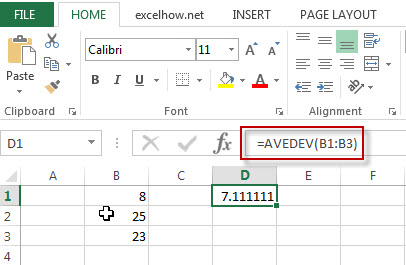# Excel AVEDEV Function

This post will guide you how to use Excel AVEDEV function with syntax and examples in Microsoft excel.

### Description

The Excel AVEDEV function returns the average of the absolute deviations of the numbers that you provided.

The AVEDEV function is a build-in function in Microsoft Excel and it is categorized as a Statistical Function.

The AVEDEV function is available in Excel 2016, Excel 2013, Excel 2010, Excel 2007, Excel 2003, Excel XP, Excel 2000, Excel 2011 for Mac.

### Syntax

The syntax of the AVEDEV function is as below:

= AVEDEV (number1, [number2],…)

Where the CHOOSE function arguments are:
Number1 -This is a required argument.  A numeric values

### Example

The below examples will show you how to use Excel AVEDEV Function to return a value from a value list based on a position value.

#1 To get the average of the absolute deviations of the numbers in cells B1:B3, just using the following excel formula: =AVEDEV(B1:B3)